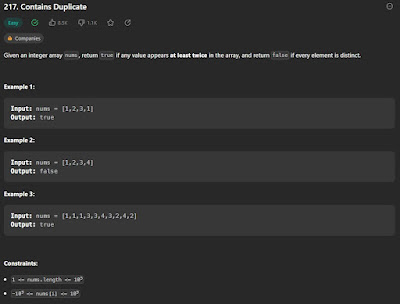## Friday, March 10, 2023

### Contains Duplicate (Leetcode)

I wrote a post roughly 2/3 years ago regarding data structures and algorithms. I thought I'd follow up with some questions I'd come across such as the famous Blind 75 Leetcode Questions and write down what my thought process to approaching the problem + run time and space complexity of the solution for the given question.

I usually solve all my questions in Python. The first of which questions we will work on is Contains Duplicate:

Regardless of the approach, we'll have to check every number in "nums" so our run time will be O(length of nums) at a minimum. We'll also assume within nums there is only one possible duplicate.

What's the most intuitive solution when we read through this? Most likely checking the current number n against all other numbers (n+1, n+2, n+3...).

We'll use the first example: we're on the first element, 1. We'll have to check 1 against 2, 1 against 3, 1 against 1. Then we'll check 2 against 3, 2 against 1, we don't have to check 2 against the initial number because we have already made that comparison before.

So we'll come up with something like the below:

Code is pretty simple, we use a for loop to iterate through nums and within that loop, we'll do another for loop to check elements of n+1 until we reach n + length(nums) - 1. This results in a O(N^2) solution because for each iteration of nums we're roughly going through it length(nums) - index times. The space complexity of this is O(1) since no extra structure is used to store anything.

Is there anything we can do to reduce the number of times we have to iterate through the list? We can perhaps use a dictionary, a key/value store. We will use the number as the key and a value depending on how many times we have come across the number. Using Example 1:
1. first iteration, the value of element at iteration 0 is 1, does the key 1 exist in the dictionary? No, we'll add it and set the value to 1
2. second iteration, the value of element at iteration 1 is 2, does the key 2 exist in the dictionary? No, we'll add it and set the value to 1
and so on until we hit iteration 4, does the key 1 exist in the dictionary? Yes! we'll return True since the key exists and this means we have found a duplicate. In another scenario if we iterate through the whole list and no duplicates are found we'll return False.

What is the complexity of the above code? O(N) time-complexity since we're only iterating through the array once (we have to create a dictionary from nums). O(N) space-complexity since we'll need to store values up to length nums in the variable my_dict.

Is there a more concise way to do this? Actually there is, we can use a structure called Sets, a set in Python is similar to a list (array) except it does not allow duplicate values.

Given a set cannot contain duplicate elements, we can compare it to the original list, if both are the same we return False, otherwise if they're not the same that must mean the nums must contain a duplicate. We arrive at something like below:

What we're doing in this case is comparing the length of nums against the length of nums turned into a set. If there are no duplicates both lengths should be equal and we'll get a value of 0 (False). Otherwise we'll get a value of 1 (True) since the length of the set nums cannot contain any duplicate values the relation between the two lengths would be something like this: length of nums >= length of set(nums).

What is the complexity of the above code? Same as the dictionary solution, as we'll only iterate through nums once and we'll have to create a set of length nums (roughly) to store values up to length nums.